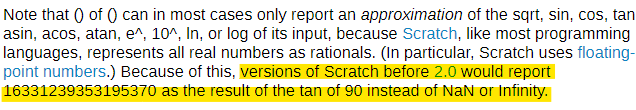# Tan(90) = 16331239353195370

Snap! for some reason returns a very large number (that is not Infinity) when I use

[scratchblocks]
([tan v] of (90))
USE BIGNUMS <✓ (­ :: grey) :: operators> :: operators //Even using this doesn't work.
[/scratchblocks]

OkConsidering that Snap! is a modified version of Scratch 1.4BYOB was a modified version of Scratch 1.4. Current versions of Snap!, however, share no code with Scratch.

[offtopic]
For me BYOB = Snap!
[/offtopic]

So, how do you explain that the tan(90) returns that number?

BYOB is not Snap! under a different name. It's an entirely different program with different capabilities.

I never said I could, just that it can't be a result of Scratch's code.

Using
[scratchblocks]
USE BIGNUMS <> :: operators
[/scratchblocks] doesn't solve the problem

aaaa

I know, you wrote that in the main post

Scratch 2.0 and 3.0 give me the correct answer.

That's because in the Scratch source code, the tangent function uses a different implementation that deals with these cases specially.

Ok. I think this should fix the problem

[scratchblocks]
(tan of ((n #) :: operators) :: operators) :: control hat
set [n v] to ((n) mod (360))
if <<(n) = [-270]> or <(n) = >>­{
report [Infinity] :: cap control
} :: control
if <<(n) = [-90]> or <(n) = >>­{
report [-Infinity] :: cap control
} :: control
report ([tan v] of (n)) :: cap control
[/scratchblocks]

You would need to change Snap's source code if you wanted to fix the problem at the lowest level though... you can't exactly ask everyone who needs the tangent function to use a custom block

Yes. But the important thing is that I have a temporary solution to the problem.

By the way, I noticed that
[scratchblocks]
([sin v] of ())
[/scratchblocks] and

[scratchblocks]
([cos v] of ())
[/scratchblocks] have the same problem as well.

Scratch fixes this with Number.prototype.toFixed, I guess you could use the round block or check for special cases.
Also, I don't see where sine has this problem, only cosine

Maybe using

[scratchblocks]
((round((­. . . :: grey) * (1e15))) / (1e15))
[/scratchblocks]
I think this script fixes the problem with those functions.

So, before we start- Snap is written js.

function getTanFromDegrees(degrees) {
return Math.tan(degrees * Math.PI / 180)
}

getTanFromDegrees(90)


This is the code you are trying to execute by running [scratchblocks]([tan v] of (90))[/scratchblocks]

First of all- Math.tan() takes radians as a parameter.
Second- 90 * Math.PI / 180 doesn't actually equal a radian of 90 degrees.
That is because Math.PI != π because, it is just an approximation
Math.PI === 3.141592653589793

Also, they just added an edge case to Scratch 2.0 and 3.0

Yes, exactly. If we measured angles in radians, we'd get a proper infinity, I believe.

I actually found a solution.

[scratchblocks]
((round(([sin v] of (n)) * (1e15))) / (1e15)) // sin
((round(([cos v] of (n)) * (1e15))) / (1e15)) // cos
((round(([sin v] of (n)) * (1e15))) / (round(([cos v] of (n)) * (1e15)))) // tan
[/scratchblocks]